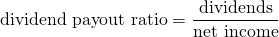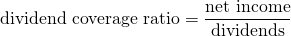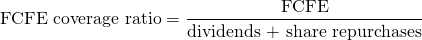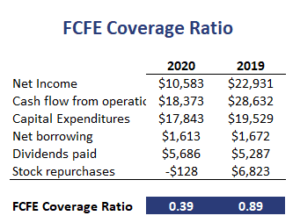# FCFE Coverage Ratio

The FCFE Coverage Ratio is a ratio that is often calculated to determine the probability of dividends continuing at the current rate or not. This ratio is typically calculated when an analyst analyzes a stock’s dividend safety. There are at least three dividend safety metrics that we can calculate. These are the dividend payout ratio, the dividend coverage ratio, and the Free Cash flow to Equity coverage ratio.

On this page, we discuss each of the dividend safety metrics that investors can calculate. We also apply the formulas using a numerical example. A dividend safety Excel calculator is available at the bottom of the page.

## Dividend payout ratio

The first ratio is the dividend payout ratio. The dividend payout ratio formula equals## Dividend coverage ratio

The second ratio is the dividend coverage ratio. Although we discuss it here as a separate ratio, most readers will immediately realize that the dividend coverage ratio formula is simply the inverse of the dividend payout ratio. As the ratio is often used in this way, we decided to report it separately, although it is strongly linked to the first ratio we discussed.## FCFE coverage ratio

The third ratio is the Free Cash flow to Equity coverage ratio. Free Cash flow to Equity (FCFE) is the cash flow available for distribution to stockholders after working capital and fixed capital needs are accounted for. The FCFE coverage ratio formula equalsThis ratio should be higher than one to be sustainable.

## FCFE coverage ratio example

The best way to use the above ratios is to compare the values for the company under consideration with the values for the industry or the market. Also, it is important to look at the trend in these ratios. Stable or increasing ratios are considered positive, whereas cuts are looked upon unfavorably. The following table applies all the measures using an Excel spreadsheet